Home Practice
For learners and parents For teachers and schools
Textbooks
Full catalogue
Pricing SupportLog in

We think you are located in United States. Is this correct?

# 7.3 Superposition of pulses

## 7.3 Superposition of pulses (ESACJ)

Two or more pulses can pass through the same medium at that same time in the same place. When they do they interact with each other to form a different disturbance at that point. The resulting pulse is obtained by using the principle of superposition.

Principle of superposition

The principle of superposition states that when two disturbance occupy the same space at the same time the resulting disturbance is the sum of two disturbances.

After pulses pass through each other, each pulse continues along its original direction of travel, and their original amplitudes remain unchanged.

Constructive interference takes place when two pulses meet each other to create a larger pulse. The amplitude of the resulting pulse is the sum of the amplitudes of the two initial pulses. This could be two crests meeting or two troughs meeting. This is shown in Figure 7.2.

Constructive interference

Constructive interference is when two pulses meet, resulting in a bigger pulse.

Destructive interference takes place when two pulses meet and result in a smaller amplitude disturbance. The amplitude of the resulting pulse is the sum of the amplitudes of the two initial pulses, but the one amplitude will be a negative number. This is shown in Figure 7.3. In general, amplitudes of individual pulses are summed together to give the amplitude of the resultant pulse.

Destructive interference

Destructive interference is when two pulses meet, resulting in a smaller pulse.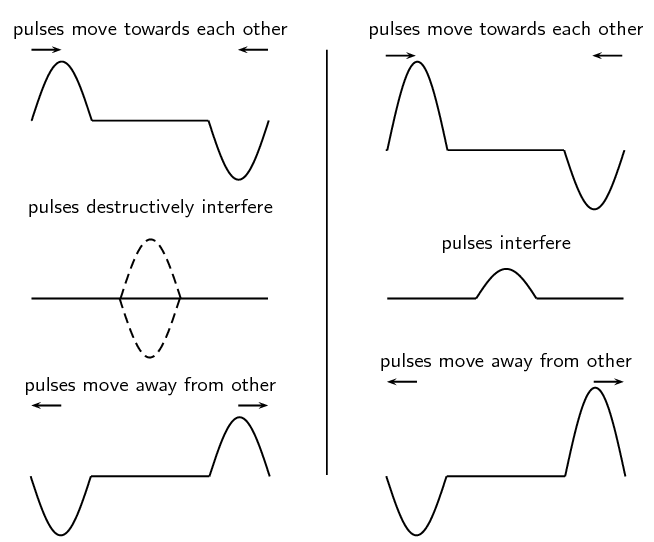Figure 7.3: Superposition of two pulses. The left-hand series of images demonstrates destructive interference, since the pulses cancel each other. The right-hand series of images demonstrate a partial cancellation of two pulses, as their amplitudes are not the same in magnitude.
temp text

## Worked example 2: Superposition of pulses

The two pulses shown below approach each other at $$\text{1}$$ $$\text{m·s^{-1}}$$. Draw what the waveform would look like after $$\text{1}$$ $$\text{s}$$, $$\text{2}$$ $$\text{s}$$ and $$\text{5}$$ $$\text{s}$$.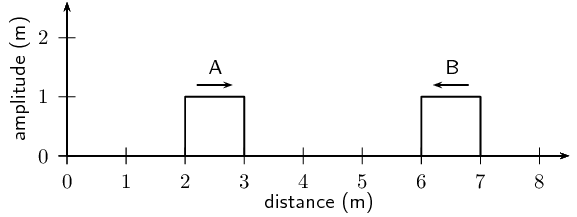### After $$\text{1}$$ $$\text{s}$$

After $$\text{1}$$ $$\text{s}$$, pulse A has moved $$\text{1}$$ $$\text{m}$$ to the right and pulse B has moved $$\text{1}$$ $$\text{m}$$ to the left.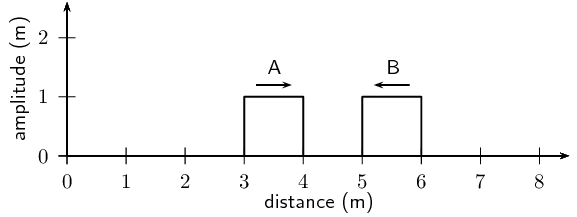### After $$\text{2}$$ $$\text{s}$$

After $$\text{1}$$ $$\text{s}$$ more, pulse A has moved $$\text{1}$$ $$\text{m}$$ to the right and pulse B has moved $$\text{1}$$ $$\text{m}$$ to the left.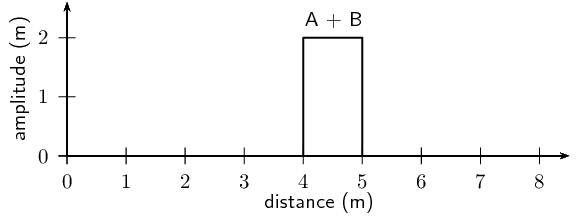### After $$\text{5}$$ $$\text{s}$$

After $$\text{5}$$ $$\text{s}$$ more, pulse A has moved $$\text{5}$$ $$\text{m}$$ to the right and pulse B has moved $$\text{5}$$ $$\text{m}$$ to the left.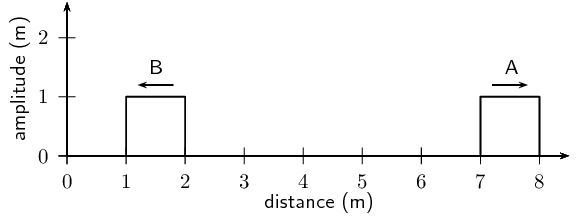temp text

## Constructive and destructive interference

### Aim

To demonstrate constructive and destructive interference

### Apparatus

Ripple tank apparatus

### Method

1. Set up the ripple tank

2. Produce a single pulse and observe what happens (you can do this any means, tapping the water with a finger, dropping a small object into the water, tapping a ruler or even using a electronic vibrator)

3. Produce two pulses simultaneously and observe what happens

4. Produce two pulses at slightly different times and observe what happens

### Results and conclusion

You should observe that when you produce two pulses simultaneously you see them interfere constructively and when you produce two pulses at slightly different times you see them interfere destructively.

## Problems involving superposition of pulses

Textbook Exercise 7.2

For the following pulse, draw the resulting wave forms after $$\text{1}$$ $$\text{s}$$, $$\text{2}$$ $$\text{s}$$, $$\text{3}$$ $$\text{s}$$, $$\text{4}$$ $$\text{s}$$ and $$\text{5}$$ $$\text{s}$$. Each pulse is travelling at $$\text{1}$$ $$\text{m·s^{-1}}$$. Each block represents $$\text{1}$$ $$\text{m}$$. The pulses are shown as thick black lines and the undisplaced medium as dashed lines.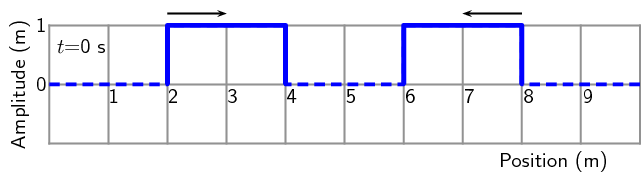Solution not yet available

For the following pulse, draw the resulting wave forms after $$\text{1}$$ $$\text{s}$$, $$\text{2}$$ $$\text{s}$$, $$\text{3}$$ $$\text{s}$$, $$\text{4}$$ $$\text{s}$$ and $$\text{5}$$ $$\text{s}$$. Each pulse is travelling at $$\text{1}$$ $$\text{m·s^{-1}}$$. Each block represents $$\text{1}$$ $$\text{m}$$. The pulses are shown as thick black lines and the undisplaced medium as dashed lines.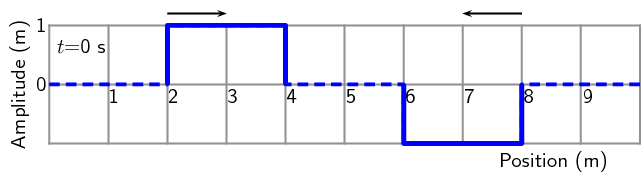Solution not yet available

For the following pulse, draw the resulting wave forms after $$\text{1}$$ $$\text{s}$$, $$\text{2}$$ $$\text{s}$$, $$\text{3}$$ $$\text{s}$$, $$\text{4}$$ $$\text{s}$$ and $$\text{5}$$ $$\text{s}$$. Each pulse is travelling at $$\text{1}$$ $$\text{m·s^{-1}}$$. Each block represents $$\text{1}$$ $$\text{m}$$. The pulses are shown as thick black lines and the undisplaced medium as dashed lines.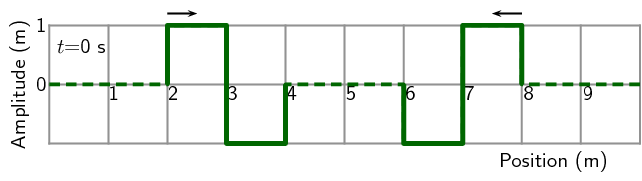Solution not yet available

For the following pulse, draw the resulting wave forms after $$\text{1}$$ $$\text{s}$$, $$\text{2}$$ $$\text{s}$$, $$\text{3}$$ $$\text{s}$$, $$\text{4}$$ $$\text{s}$$ and $$\text{5}$$ $$\text{s}$$. Each pulse is travelling at $$\text{1}$$ $$\text{m·s^{-1}}$$. Each block represents $$\text{1}$$ $$\text{m}$$. The pulses are shown as thick black lines and the undisplaced medium as dashed lines.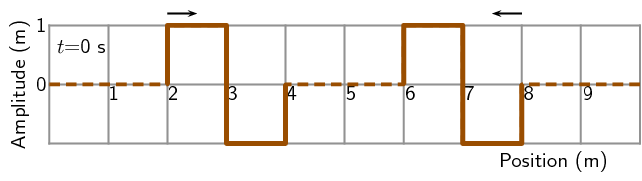Solution not yet available

For the following pulse, draw the resulting wave forms after $$\text{1}$$ $$\text{s}$$, $$\text{2}$$ $$\text{s}$$, $$\text{3}$$ $$\text{s}$$, $$\text{4}$$ $$\text{s}$$ and $$\text{5}$$ $$\text{s}$$. Each pulse is travelling at $$\text{1}$$ $$\text{m·s^{-1}}$$. Each block represents $$\text{1}$$ $$\text{m}$$. The pulses are shown as thick black lines and the undisplaced medium as dashed lines.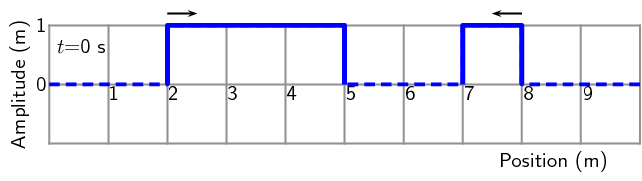Solution not yet available

For the following pulse, draw the resulting wave forms after $$\text{1}$$ $$\text{s}$$, $$\text{2}$$ $$\text{s}$$, $$\text{3}$$ $$\text{s}$$, $$\text{4}$$ $$\text{s}$$ and $$\text{5}$$ $$\text{s}$$. Each pulse is travelling at $$\text{1}$$ $$\text{m·s^{-1}}$$. Each block represents $$\text{1}$$ $$\text{m}$$. The pulses are shown as thick black lines and the undisplaced medium as dashed lines.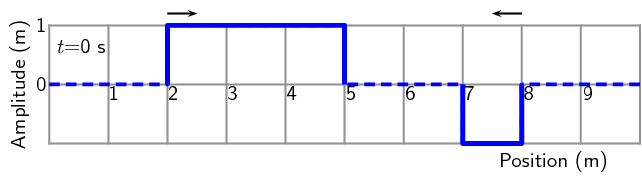Solution not yet available

What is superposition of waves?

Solution not yet available

What is constructive interference?

Solution not yet available

What is destructive interference?

Solution not yet available